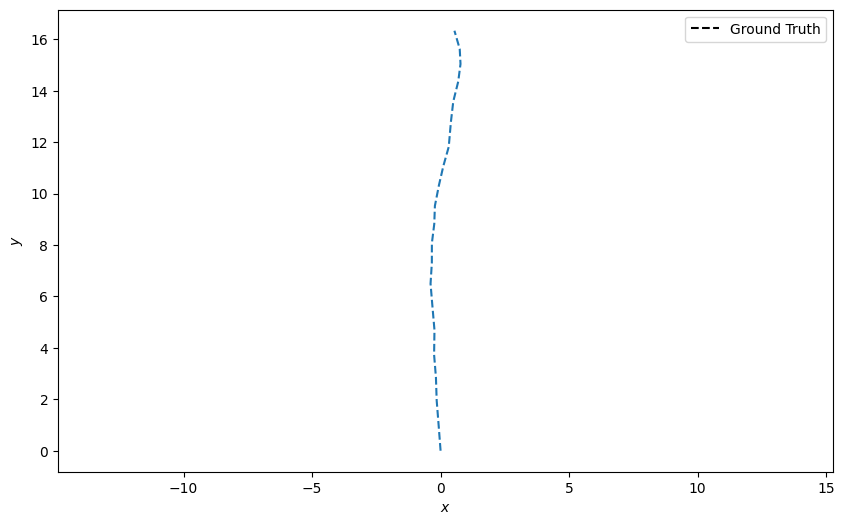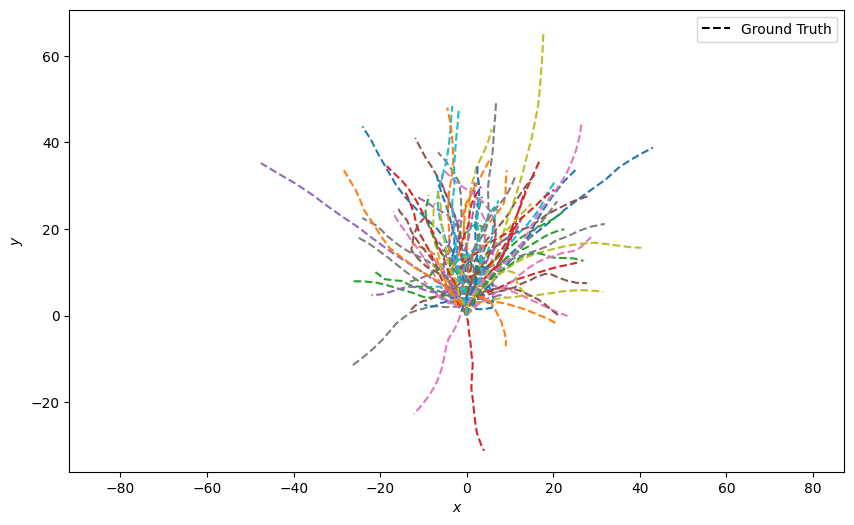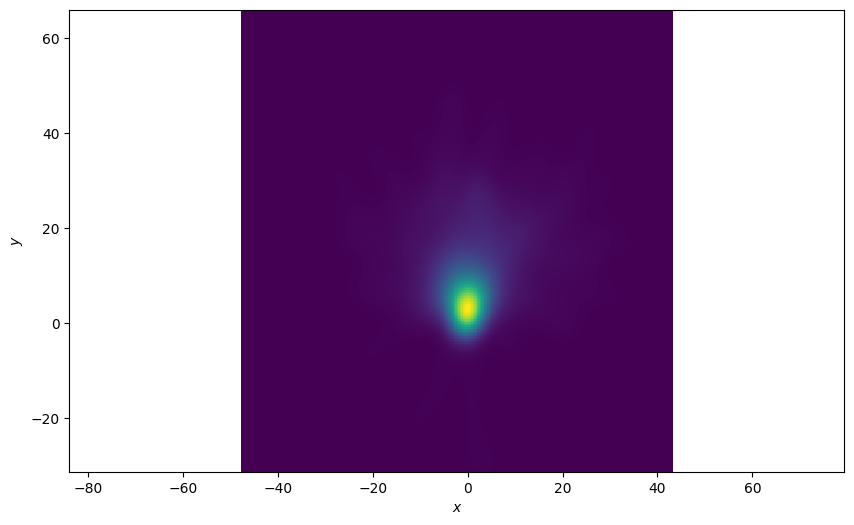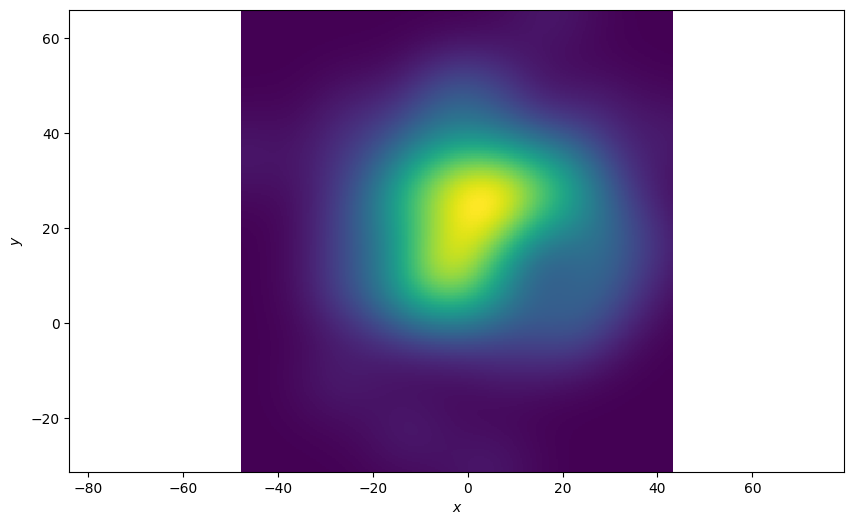# Density Plot Example

This example looks at how to plot 2d density plots. The Plotter().plot_density function can be used to plot any number of `StateMutableSequence` objects. StateMutableSequences are just a container for a number of states, examples include tracks and ground truth paths. The examples below show how to plot ground truth paths (as they’re easy to generate). The function can be used to analyse large data sets.

Set the imports and set the start time

```from datetime import datetime, timedelta
from matplotlib import animation

from stonesoup.types.groundtruth import GroundTruthPath, GroundTruthState
from stonesoup.models.transition.linear import CombinedLinearGaussianTransitionModel, \
ConstantVelocity
from stonesoup.plotter import Plotter

start_time = datetime.now()
```

## Generate the State Sequences to Plot

In these examples we’re going plot ground-truth as that is the easiest to generate. An simple function has been created to ground truth

```def generate_ground_truth_path(initial_state, num_steps=20, motion_model_noise=0.01):

transition_model = CombinedLinearGaussianTransitionModel(
[ConstantVelocity(motion_model_noise), ConstantVelocity(motion_model_noise)])

ground_truth = GroundTruthPath([GroundTruthState(initial_state, timestamp=start_time)])

for k in range(0, num_steps):
ground_truth.append(GroundTruthState(
transition_model.function(ground_truth[k], noise=True,
time_interval=timedelta(seconds=1)),
timestamp=start_time+timedelta(seconds=k+1)))

return ground_truth
```

Create one ground truth path starting at the origin (0,0) and plot it

```n_time_steps = 20
truth = generate_ground_truth_path(initial_state=[0, 0, 0, 1], num_steps=n_time_steps)

plotter = Plotter()
plotter.plot_ground_truths(truth, [0, 2])
```Generate 100 ground truth paths and plot them all at once. This looks quite messy

```truths = [generate_ground_truth_path(initial_state=[0, 0, 0, 1],
num_steps=n_time_steps,
motion_model_noise=0.1)
for _ in range(100)]

plotter = Plotter()
plotter.plot_ground_truths(set(truths), [0, 2])
```### Density Plot of All States

Plot a 2d density plot for all the states in the ground-truth. This is clearer, we can see a clear concentration around the origin where all the tracks start

```plotter = Plotter()
plotter.plot_density(truths, index=None)
```### Plot of the Last State of the Ground Truths

The function allows you to pick an index of the state sequence (ground truth in this example) to plot. In this example we’re only interested in the final state of the sequences. An index of ‘-1’ is the last state in the sequence. The resultant plot is much more spread out

```plotter = Plotter()
plotter.plot_density(truths, index=-1)
```### Plot each state over time

Plot the density at each time-step and see how the density plot evolves. Define an animation update function.

```def update(i):
plotter.ax.clear()
plotter.plot_density(truths, index=i)
return plotter.ax
```

Plot the densities over time.

```plotter = Plotter()
animation.FuncAnimation(plotter.fig, update, frames=range(1, n_time_steps))
```